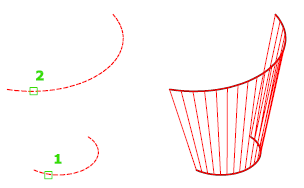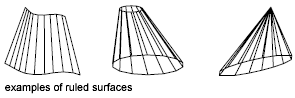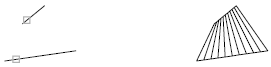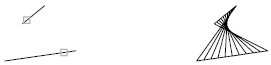# RULESURF (Command)

Creates a mesh that represents the surface between two lines or curves.

Find

Select two edges that define the mesh. The edges can be lines, arcs, splines, circles, or polylines. If one of the edges is closed, then the other edge must also be closed. You can also use a point as one edge for either an open or a closed curve.The MESHTYPE system variable sets which type of mesh is created. Mesh objects are created by default. Set the variable to 0 to create legacy polyface or polygon mesh.

For closed curves, the selection does not matter. If the curve is a circle, the ruled mesh begins at the 0-degree quadrant point, as determined by the current X axis plus the current value of the SNAPANG system variable. For closed polylines, the ruled mesh starts at the last vertex and proceeds backward along the segments of the polyline. Creating a ruled mesh between a circle and a closed polyline can be confusing. Substituting a closed semicircular polyline for the circle might be preferable.The ruled mesh is constructed as a 2 by N polygon mesh. RULESURF places half the mesh vertices at equal intervals along one defining curve, and the other half at equal intervals along the other curve. The number of intervals is specified by the SURFTAB1 system variable. It is the same for each curve; therefore, the distance between the vertices along the two curves differs if the curves are of different lengths.

The N direction of the mesh is along the boundary curves. If both boundaries are closed, or if one is closed and the other is a point, the resulting polygon mesh is closed in the N direction and N equals SURFTAB1. If both boundaries are open, N equals SURFTAB1 + 1, because division of a curve into n parts requires n + 1 tabulations.

The 0,0 vertex of the mesh is the endpoint of the first selected curve nearest the point you used to select that curve.

Selecting objects at the same ends creates a polygon mesh.Selecting objects at opposite ends creates a self-intersecting polygon mesh.The following prompts are displayed.

First defining curve

Specifies an object and start point for the new mesh object.

Second defining curve

Specifies an object and start point for the sweep of the new mesh object.